## 发布标记

### 标记概述

• 使用发布选项卡上的格式设置按钮和下拉菜单设置文件格式。此方法可自动为您插入文本标记。

• 从右键点击菜单的插入文本标记列表中选择标记。

• 直接在注释中键入标记。

• 注释符号 (%) 后面的空格通常确定随后的文本的格式。

• 开始新标记通常需要前面的空注释行，如示例中所示。

• 标记仅在紧跟有分节符的注释中有效。

%% SECTION TITLE % DESCRIPTIVE TEXT 
%%% SECTION TITLE WITHOUT SECTION BREAK % DESCRIPTIVE TEXT

% _ITALIC TEXT_
% *BOLD TEXT*
% |MONOSPACED TEXT|
% Trademarks: % TEXT(TM)
% TEXT(R)

%% Bulleted List % % * BULLETED ITEM 1 % * BULLETED ITEM 2 %
%% Numbered List % % # NUMBERED ITEM 1 % # NUMBERED ITEM 2 %

%% % % PREFORMATTED % TEXT % 
%% MATLAB(R) Code % % for i = 1:10 % disp x % end % 

% % <include>filename.m</include> %

% % <<FILENAME.PNG>> %

snapnow;
LaTeX 方程
%% Inline Expression % $x^2+e^{\pi i}$ 
%% Block Equation % % $$e^{\pi i} + 1 = 0$$ % 

% <https://www.mathworks.com MathWorks>
% <matlab:FUNCTION DISPLAYED_TEXT> 
HTML 标记
% % <html> % <table border=1><tr> % <td>one</td> % <td>two</td></tr></table> % </html> % 
LaTeX 标记
%% LaTeX Markup Example % <latex> % \begin{tabular}{|r|r|} % \hline $n$&$n!$\\ % \hline 1&1\\ 2&2\\ 3&6\\ % \hline % \end{tabular} % </latex> %

### 节和节标题

%% Vector Operations % You can perform a number of binary operations on vectors. %% A = 1:3; B = 4:6; %% Dot Product % A dot product of two vectors yields a scalar. % MATLAB has a simple command for dot products. s = dot(A,B); %% Cross Product % A cross product of two vectors yields a third % vector perpendicular to both original vectors. % Again, MATLAB has a simple command for cross products. v = cross(A,B);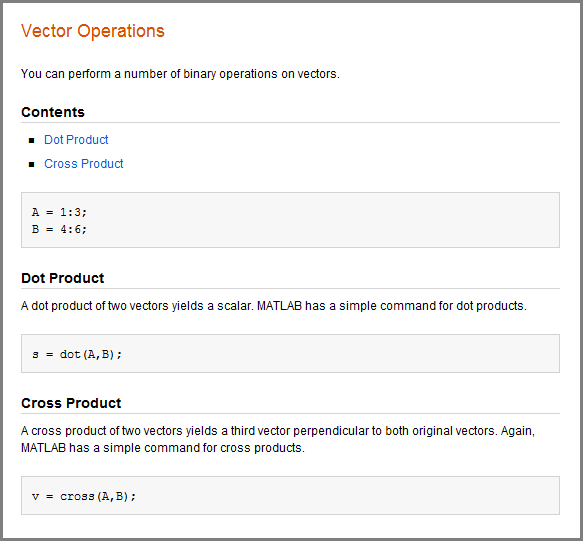### 文本格式设置

%% Calculate and Plot Sine Wave % _Define_ the *range* for |x|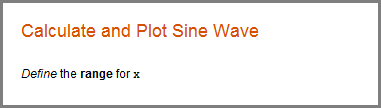#### 商标符号

%% Basic Matrix Operations in MATLAB(R) % This is a demonstration of some aspects of MATLAB(R) % and the Symbolic Math Toolbox(TM).### 项目符号和编号列表

MATLAB 允许在注释中使用项目符号列表和编号列表。您可以使用此语法生成项目符号列表和编号列表。

%% Two Lists % % * ITEM1 % * ITEM2 % % # ITEM1 % # ITEM2 %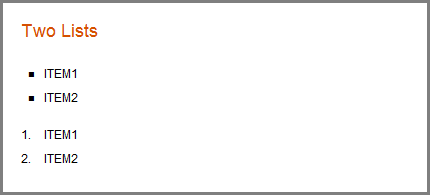### 文本和代码块

#### 预设格式的文本

%% % Many people find monospaced text easier to read: % % A dot product of two vectors yields a scalar. % MATLAB has a simple command for dot products.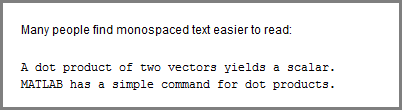#### 语法高亮的示例代码

%% % % for i = 1:10 % disp(x) % end %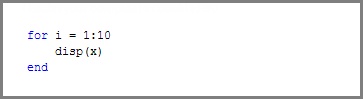### 外部文件内容

%% External File Content Example % This example includes the file contents of sine_wave.m into published % output. % % <include>sine_wave.m</include> % % The file content above is properly syntax highlighted.### 外部图形

%% % % <<FILENAME.PNG>> % 

MATLAB 要求 FILENAME.PNG 是从输出位置到您的外部图像的相对路径，或者是完全限定 URL。最好将您的图像保存在 MATLAB 发布其输出的同一文件夹中。例如，MATLAB 将 HTML 文档发布到子文件夹 html。将您的图像文件保存在同一子文件夹中。您可以通过更改发布配置设置来更改输出文件夹。在 MATLAB Online™ 中，将您的图像文件保存到位于根文件夹下的 Published 文件夹中。

#### 使用 surf(peaks) 的外部图形示例

saveas(surf(peaks),'surfpeaks.jpg');

1. 在当前文件夹中创建一个名为 html 的子文件夹。

2. 通过在命令行窗口中运行此代码创建 surfpeaks.jpg

saveas(surf(peaks),'html/surfpeaks.jpg');

3. 将此 MATLAB 代码发布为 HTML。

%% Image Example % This is a graphic: % % <<surfpeaks.jpg>> %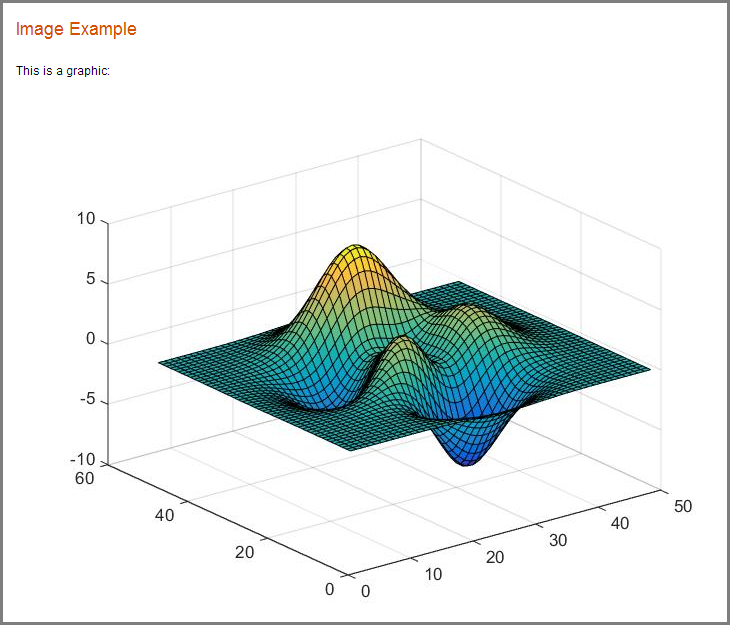#### 输出文件格式的有效图像类型

docpng

htmlpng

latexpngepsc2

pdfbmp

bmpjpg

pptpng

xmlpng

### 图像快照

%% Scale magic Data and Display as Image for i=1:3 imagesc(magic(i)) snapnow; end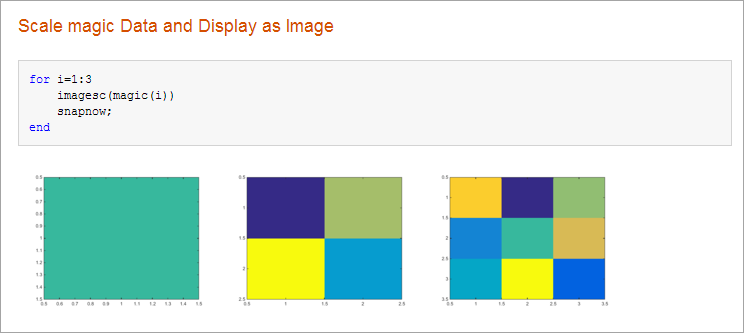### LaTeX 方程

#### 行内 LaTeX 表达式

• 所有发布输出类型都支持 LaTeX 表达式，但 Microsoft PowerPoint® 除外。

• MATLAB 发布支持标准 LaTeX 数学模式的指令。不支持文本模式的指令或需要额外包的指令。

%% LaTeX Inline Expression Example % % This is an expression: $x^2+e^{\pi i}$. It is % inline with the text.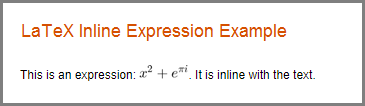#### LaTeX 行间方程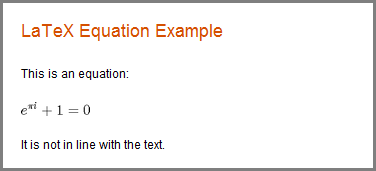### 超链接

#### 静态超链接

%% % For more information, see our web site: % <https://www.mathworks.com MathWorks>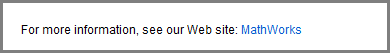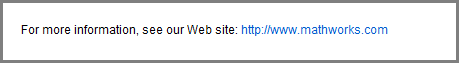#### 动态超链接

%% Recycling Preference % Click the preference you want: % % <matlab:recycle('off') Disable recycling> % % <matlab:recycle('on') Enable recycling>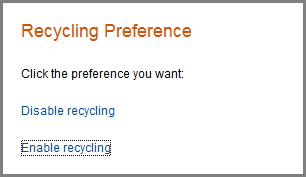%% % See the % <matlab:edit(fullfile(matlabroot,'toolbox','matlab','codetools','publish.m')) code> % for the publish function.%% % See the help for the <matlab:doc('publish') publish> function.### HTML 标记

%% HTML Markup Example % This is a table: % % <html> % <table border=1><tr><td>one</td><td>two</td></tr> % <tr><td>three</td><td>four</td></tr></table> % </html> %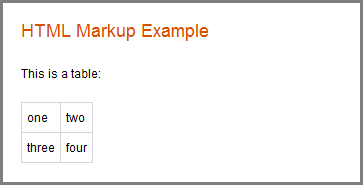disp('<html><table><tr><td>1</td><td>2</td></tr></table></html>')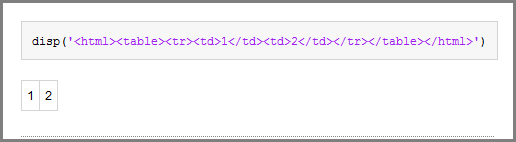### LaTeX 标记

%% LaTeX Markup Example % This is a table: % % <latex> % \begin{tabular}{|c|c|} \hline % $n$ & $n!$ \\ \hline % 1 & 1 \\ % 2 & 2 \\ % 3 & 6 \\ \hline % \end{tabular} % </latex> 

% This LaTeX was auto-generated from MATLAB code. % To make changes, update the MATLAB code and republish this document. \documentclass{article} \usepackage{graphicx} \usepackage{color} \sloppy \definecolor{lightgray}{gray}{0.5} \setlength{\parindent}{0pt} \begin{document} \section*{LaTeX Markup Example} \begin{par} This is a table: \end{par} \vspace{1em} \begin{par} \begin{tabular}{|c|c|} \hline $n$ & $n!$ \\ \hline 1 & 1 \\ 2 & 2 \\ 3 & 6 \\ \hline \end{tabular} \end{par} \vspace{1em} \end{document}

MATLAB 包括使用 LaTeX 程序编译此文件所需的任何其他标记。